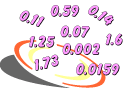In the last lesson, you were introduced to decimal numbers. Decimal places change by a factor of 10. For example, let's look at the number '3247.8956' below.

 3 x 1000 thousands 2 x 100 hundreds 4 x 10 tens 7 x 1 ones 8 x 0.1 tenths 9 x 0.01 hundredths 5 x 0.001 thousandths 6 x 0.0001 ten-thousandths

A decimal number can have a whole-number part and a fractional part.

 Mixed Number - Expanded Form - Decimal Form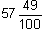= (5 x 10) + ( 7 x 1) + (4 x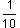) + (9 x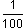) = 57.49 - whole-number part - - fractional part -

In this lesson, you will learn how to read and write decimals. You may use our Place Value and Decimals Chart (PDF) as a visual reference for the examples presented in this lesson.

Example 1: Write each mixed number as a decimal.

 Mixed Number Decimal52.3000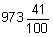973.4100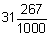31.2670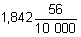1842.0056

Example 2: Write each phrase as a mixed number and as a decimal.

 phrase mixed number decimal five and three tenths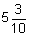5.300000 forty-nine and one hundredth49.010000 two hundred sixteen and two hundred thirty-one thousandths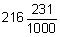216.231000 nine thousand, ten and three hundred fifty-nine ten-thousandths9,010.035900 seventy-six thousand, fifty-three and forty-seven hundred-thousandths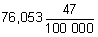76,053.000470 two hundred twenty-nine thousand and eighty-one millionths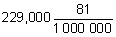229,000.000081

Look at the mixed numbers in the examples above. You will notice that the denominator of the fractional part is a factor of 10, making it is easy to convert to a decimal. Let's look at some examples in which the denominator is not a factor of 10.

Example 3: Write each mixed number as a decimal.

Analysis: A fraction bar tells us to divide. In order to do this, we must convert or change the fractional part of each mixed number to decimal digits. We will do this by dividing the numerator of each fraction by its denominator.

 Mixed Number Fractional Part Decimal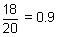6.90009.7200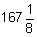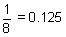167.1250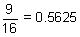149.5625Alternate Method: It should be noted that some of the fractions above could have been converted to decimals using equivalent fractions. For example: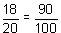Example 4: When asked to write two hundred thousandths as a decimal, three students gave three different answers as shown below. Which student had the correct answer?

Student 1: 200,000.

Student 2: 0.200

Student 3: 0.00002

Analysis: Let's use our place value chart to help us analyze this problem.

 PLACE VALUE AND DECIMALS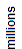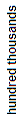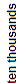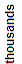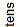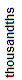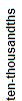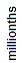Student 1 2 0 0 0 0 0 . Student 2 0 . 2 0 0 Student 3 0 . 0 0 0 0 2Let's look at the expanded form of each decimal to help us find the correct answer.

 Student Number Fraction Expanded Form Phrase 1 200,000.00000 2 x 100,000 two hundred thousand 2 0.20000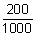two hundred thousandths 3 0.00002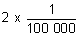two hundred-thousandthsAs you can see, decimals are named by the place of the last digit. Notice that in Example 4, the answer given by Student 3 was two hundred-thousandths. This phrase has a hyphen in it. The hyphen is an important piece of information that helps us read and write decimals. Let's look at some more examples.

Example 5: Write each phrase as a decimal.

 phrase analysis fraction decimal three hundred ten thousandths 310 thousandths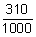0.310 three hundred ten-thousandths 300 ten-thousandths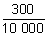0.0300

Example 6: Write each phrase as a decimal.

 phrase analysis fraction decimal eight hundred thousandths 800 thousandths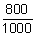0.800 eight hundred-thousandths 8 hundred-thousandths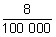0.00008

Example 7: Write each phrase as a decimal.

 phrase analysis fraction decimal seven hundred millionths 700 millionths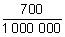0.000700 seven hundred-millionths 7 hundred-millionths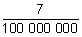0.00000007

In Examples 5 through 7, we were asked to write phrases as decimals. Some of the words in the phrase indicate the place-value positions, and other words in the phrase indicate the digits to be used. Now let's look at some examples in which we write these kinds of decimals using words.

Example 8: Write each decimal using words.

 decimal analysis phrase 0.110 110 thousandths one hundred ten thousandths 0.0100 100 ten-thousandths one hundred ten-thousandths

Example 9: Write each decimal using words.

 decimal analysis phrase 0.400 400 thousandths four hundred thousandths 0.00004 4 hundred-thousandths four hundred-thousandths

Example 10:  Write the following decimal using words. Roll your mouse over each digit for help.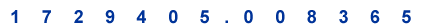Answer: The decimal 1,729,405.008365 is written as:

one million, seven hundred twenty-nine thousand, four hundred five and eight thousand, three hundred sixty-five millionths

Summary: You learned how to read and write decimals in this lesson. When writing a mixed number as a decimal, the fractional part must be converted to decimal digits. Decimals are named by the place of the last digit. The hyphen is an important indicator when reading and writing decimals. When writing a phrase as a decimal, some of the words indicate the place-value positions, and other words indicate the digits to be used.

### Exercises

In Exercises 1 and 2, click once in an ANSWER BOX and type in your answer; then click ENTER. After you click ENTER, a message will appear in the RESULTS BOX to indicate whether your answer is correct or incorrect. To start over, click CLEAR.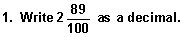ANSWER BOX:       RESULTS BOX: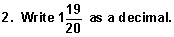ANSWER BOX:        RESULTS BOX:
 In Exercises 3 through 5, read each question below. Select your answer by clicking on its button. Feedback to your answer is provided in the RESULTS BOX. If you make a mistake, choose a different button.
 3. Which of the following is equal to seven hundred five thousand and eighty-nine ten-thousandths?700.005.089705,000.089705,000.0089705,000.00089 RESULTS BOX:
 4. Which of the following is equal to 9,842.1039?nine thousand, eight hundred, forty two and one thousand, thirty-nine millionthsnine thousand, eight hundred, forty-two and one thousand, thirty-nine ten-thousandthsnine thousand, eight hundred, forty-two and one thousand, thirty-nine thousandthsNone of the above. RESULTS BOX:
 5. Which of the following is equal to five hundred-thousandths?500,0000.5000.00050.00005 RESULTS BOX: